## Homework 8

1. Calculate thephase shift for the spherical potential well for both and attractive and repulsive potential.

2. Calculate thephase shift for a hard sphereforand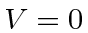for. What are the limits forlarge and small?

3. Show that at large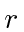, the radial flux is large compared to the angular components of the flux for wave-functions of the form.

4. Calculate the difference in wavelengths of the 2p to 1s transition in Hydrogen and Deuterium. Calculate the wavelength of the 2p to 1s transition in positronium.

5. Tritium is a unstable isotope of Hydrogen with a proton and two neutrons in the nucleus. Assume an atom of Tritium starts out in the ground state. The nucleus (beta) decays suddenly into that of He. Calculate the probability that the electron remains in the ground state.

6. A hydrogen atom is in the state. What are the possible energies that can be measured and what are the probabilities of each? What is the expectation value of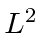? What is the expectation value of? What is the expectation value of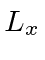?

7. What is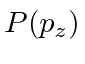, the probability distribution offor the Hydrogen energy eigenstate? You may find the expansion ofin terms of Bessel functions useful.

8. The differential equation for the 3D harmonic oscillatorhas been solved in the notes, using the same techniques as we used for Hydrogen. Use the recursion relations derived there to write out the wave functions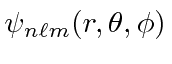for the three lowest energies. You may write them in terms of the standardbut please write out the radial parts of the wavefunction completely. Note that there is a good deal of degeneracy in this problem so the three lowest energies actually means 4 radial wavefunctions and 10 total states. Try to write the solutionsand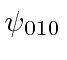in terms of the solutions in cartesian coordinates with the same energy.

Jim Branson 2013-04-22